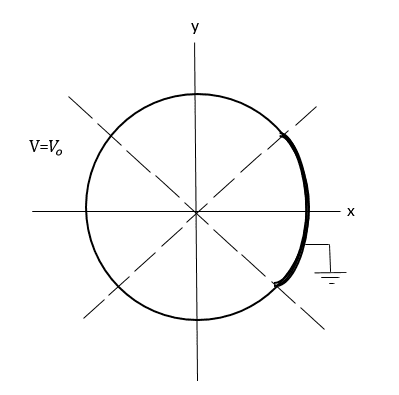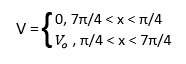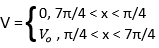# Calculating the electric potential

physicsisfun0

## Homework StatementWe have the cross section of a metal pipe that has been split into four sections. Three of the sections have a constant electric potential, Vo. The fourth section is grounded so electric potential is zero. We are looking for electric potential inside and outside of the pipe.

## Homework Equations

For electric potential I have:
V = ao + boln(s) +Σ(sv(avcos(vφ) + bosin(vφ)) + s-v(cvcos(vφ) + dvsin(vφ))
We also know boundary conditions:## The Attempt at a Solution

(I think this next part is right)
And electric potential inside becomes:
V = ao + boln(s) +Σ(sv(avcos(vφ) + bosin(vφ))
And electric potential outside becomes:
V = ao + boln(s) +Σs-v(cvcos(vφ) + dvsin(vφ))

There is also symmetry along the x-axis so we can ignore the sin(vφ) contribution:
V = ao + boln(s) +Σ(sv(avcos(vφ))
V = ao + boln(s) +Σ(s-v(avcos(vφ))

Do I need to solve for ao and bo? How would I use the boundary conditions?

#### Attachments

Homework Helper
Gold Member
Welcome to PF!Note that the first equation above implies that ##7 \pi / 4## is less than ##\pi/4##. But, the intention is clear.

V = ao + boln(s) +Σ(sv(avcos(vφ))
V = ao + boln(s) +Σ(s-v(avcos(vφ))
OK, but it might be confusing to use the same notation for the coefficients for the two different regions.
Do I need to solve for ao and bo? How would I use the boundary conditions?
For the region inside the pipe, note that s can be zero. What does that tell you about the value of bo for the inside region?
For the region outside the pipe, note that s can become arbitrarily large. What does that tell you about bo for the outside region?

To find ao, set s equal to the radius of the pipe in the above two equations. For each equation, integrate both sides of the equation with respect to φ from 0 to 2π. The boundary condition tells you V as a function of φ for the integration of the left side.

To find other coefficients an, multiply both sides of the equation by cos(nφ) before integrating.

#### Attachments

•Delta2